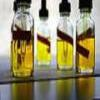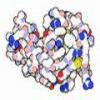#### You may also like### Mathematical Issues for Chemists

A brief outline of the mathematical issues faced by chemistry students.### Reaction Rates

Explore the possibilities for reaction rates versus concentrations with this non-linear differential equation### Mixed up Mixture

Can you fill in the mixed up numbers in this dilution calculation?

# Molecular Sequencer

##### Age 14 to 18Challenge Level

Methanal, ethanal, propanal and butanal form the first four compounds in a sequence of alkyl aldehydes with molecular formulae

CH$_2$O, C$_2$H$_4$O, C$_3$H$_6$O, C$_4$H$_8$O

What would be the molecular formula for pentanal and hexanal?

What would be the general formula for the $n$th molecule in the sequence? What masses could it have, taking into account these common isotopes of C, H and O:

$^{16}$O $99.76\%$, $^{17}$O $0.04\%$, $^{18}$O $0.2\%$

$^{12}$C $98.9\%$, $^{13}$C $1.1\%$

$^1$H $99.99\%$, $^2$H $0.01\%$

The relative abundance of the lightest form of one particular type of alkyl aldehyde is almost exactly $8$ times that of its next lightest form. Can you work out its molecular formula?